# NCERT Exemplar Problems and their Solutions

Reviewed By:
Krishna Kant Majee
M.Sc., B.Ed.

### Chapter 4-Carbon and Its Compounds-Long Answers

Q.47. A salt X is formed and a gas is evolved when ethanoic acid reacts with sodium hydrogen carbonate. Name the salt X and the gas evolved. Describe an activity and draw the diagram of the apparatus to prove that the evolved gas is the one which you have named. Also, write the chemical equation of the reaction involved.
Ethanoic $(CH_{3}COOH)$ acid reacts with sodium hydrogen carbonate (NaHCO3) to form sodium ethanoate $(CH_{3}COONa)$, water and carbon dioxide gas. Hence salt X is sodium ethanoate and the gas evolved is $CO_{2}$. The chemical equation of the reaction involved is:
$CH_{3}COOH + NaHCO_{3} → CH_{3}COONa + H_{2}O + CO_{2}$
Activity for observing the gas evolved in above reaction: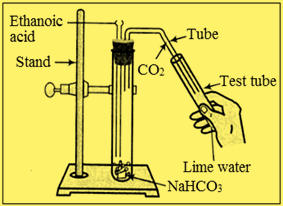Set up the apparatus and take sodium hydrogen carbonate in test tube. Add ethanoic acid to it and observe brisk effervescence of carbon dioxide $(CO_{2})$ gas. Now pass this gas through freshly prepared lime water $(Ca(OH)_{2}).$ It will turn milky due to the formation of insoluble calcium carbonate $(CaCO_{3}).$
$Ca(OH)_{2} + CO_{2} → CaCO_{3} + H_{2}O$
(Milky)
Q.48. (a) What are hydrocarbons? Give examples.
(b) Give the structural differences between saturated and unsaturated hydrocarbons with two examples each.
(c) What is functional group? Give examples of four different functional groups.
(a) Compounds which are formed from carbon and hydrogen atoms are called as hydrocarbons. Some common examples of hydrocarbons are methane $(CH_{4})$, ethane $(C_{2}H_{6})$, ethene $(CH_{2} = CH_{2})$, ethyne $(C_{2}H_{2})$, cyclohexane $(C_{6}H_{12})$ and benzene $(C_{6}H_{5})$.
Examples of saturated hydrocarbons: Methane $(CH_{4})$, Ethane $(CH_{3} – CH_{3})$
Examples of unsaturated hydrocarbons: Ethene $(H_{2}C = CH_{2}), Ethyne (HC ≡CH) (c) An atom or group of atoms that is responsible for characteristics physical and chemical properties for organic compound is found is called as functional group. Examples of four functional groups are:  Name of functional group Formula of functional group Alcohol ─ OH Carboxylicacid COOH Aldehyde CHO Ketone >C=O Q.49. Name the reaction which is commonly used in the conversion of vegetable oils to fats. Explain the reaction involved in detail. Answer. The reaction that is used to convert vegetable oil to fat is called as hydrogenation reaction. Vegetable oils contain unsaturated hydrocarbons which can exhibit addition reaction with hydrogen to form saturated hydrocarbons or fats. The reaction occurs in the presence of metal catalyst like finely divided nickel or palladium at 200 oC and forms saturated vegetable fats. The hydrogenation reaction is an industrial method for the manufacturing of vanaspati ghee from vegetable oil.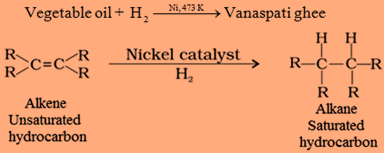Here, R is any alkyl group which usually contains a large number of carbon atoms in vegetable oils. Q.50. (a) Write the formula and draw electron dot structure of carbon tetrachloride. (b) What is saponification? Write the reaction involved in this process. Answer. (a) The molecular formula of carbon tetrachloride is$CCl_{4}$and electron dot structure is as below.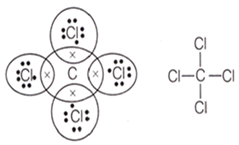Carbon tetrachloride (b) Alkaline hydrolysis of an ester in the presence of a strong base like sodium hydroxide is called as Saponification. It results the formation of corresponding salts of carboxylic acids and alcohol.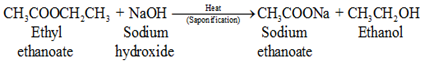Q.51. Esters are sweet-smelling substances and are used in making perfumes. Suggest some activity and the reaction involved for the preparation of an ester with well labelled diagram. Answer. Activity: Take a test tube and add 1 mL ethanol and 1 mL glacial acetic acid with a few drops of concentrated sulphuric acid. Now heat the solution in water bath for at least five minutes. Pour the content into a beaker containing water and smell the mixture. Sweet smell of ester would be observed.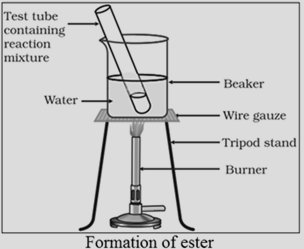Reaction of ethanoic acid$(CH_{3}COOH)$and ethanol$(CH_{3}CH_{2}OH)$in the presence of concentrated sulphuric acid (H2SO4) forms ethyl ethanoate$(CH_{3}COOC_{2}H_{5})$with water.$CH_{3}COOH + CH_{3}CH_{2}OH → CH_{3}COOC_{2}H_{5} + H_{2}O$Q.52. A compound C (molecular formula,$C_{2}H_{4}O_{2})$reacts with Na metal to form a compound R and evolves a gas which burns with a pop sound. Compound C on treatment with an alcohol A in the presence of an acid forms a sweet smelling compound S (molecular formula,$C_{3}H_{6}O_{2})$. On addition of NaOH to C, it also gives R and water. S on treatment with NaOH solution gives back R and A. Identify C, R, A, S and write down the reactions involved. Answer. Compound C with molecular formula$C_{2}H_{4}O_{2}$contains two oxygen atoms so it can be either ester or carboxylic acid. Since it reacts with sodium metal to form compound R and evolves a gas which burns with pop sound, therefore it should be a carboxylic acid which forms sodium alkanoate and hydrogen gas with sodium metal.$2CH_{3}COOH + 2Na → 2CH_{3}COONa + H_{2} ↑$The gas which burns with pop sound is hydrogen gas. Reaction of ethanoic acid with alcohol in the presence of an acid (Conc. H2SO4) forms sweet smelling ester. So compound S that is formed due to reaction of ethanoic acid and methanol (A) is methyl ethanoate with molecular formula C3H6O2 and structural formula$CH_{3}COOCH_{3}$. Hence compound C = Ethanoic acid$(CH_{3}COOH)$, R = Sodium ethanoate$(CH_{3}COONa)$, A = Methanol$(CH_{3}OH)$and S = Methyl merhanoate$(CH_{3}COOCH_{3})$Q.53. Look at the figure and answer the following questions.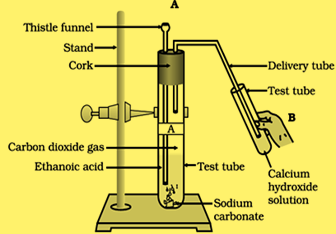(a) What change would you observe in the calcium hydroxide solution taken in tube B? (b) Write the reaction involved in test tubes A and B respectively. (c) If ethanol is given instead of ethanoic acid, would you expect the same change? (d) How can a solution of lime water be prepared in the laboratory? Answer. (a) Calcium hydroxide solution in test tube B will react with carbon dioxide gas and turns milky due to the formation of insoluble calcium carbonate$(CaCO_{3})$. (b) Test tube A:$Na_{2}CO_{3} + 2CH_{3}COOH → 2CH_{3}COONa + CO_{2} + H_{2}O$Test tube B:$Ca(OH)_{2} + CO_{2} → CaCO_{3} + H_{2}O$(c) Ethanol is weakly acidic and cannot decompose$Na_{2}CO_{3}$to give CO2 gas. (d) Lime water (calcium hydroxide) is usually prepared by the reaction of calcium oxide (quick lime) with water. The supernatent liquid thus formed is decanted or filtered off to get a clear solution of lime water. Q.54. How would you bring about the following conversions? Name the process and write the reaction involved. (a) Ethanol to ethene (b) Propanol to propanoic acid Write the reactions. Answer. (a) Ethanol can be converted to ethene by dehydration of ethanol in the presence of concentrated sulphuric acid at 170 °C temperature. The reaction is named as dehydration reaction.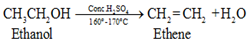(b) Propanol can be converted to propanoic acid by oxidation in the presence of alkaline potassium permanganate and heat. It is named as oxidation reaction.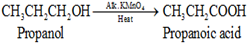Q.55. Draw the possible isomers of the compound with molecular formula$C_{3}H_{6}O$and also give their electron dot structures. Answer. Five isomers possible for the molecular formula$C_{3}H_{6}O$are listed below.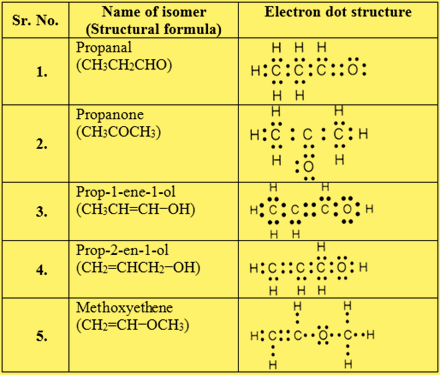Q.56. Explain the given reactions with the examples. (a) Hydrogenation reaction (b) Oxidation reaction (c) Substitution reaction (d) Saponification reaction (e) Combustion reaction Answer. (a) Hydrogenation reaction: It can be defined as the addition of hydrogen on unsaturated molecule to make saturated one. For example hydrogenation of ethene forms ethane in the presence of metal catalyst like Nickel at high temperature. (b) Oxidation reaction: Oxidation reaction is addition of oxygen with the help of some oxidizing agent like alkaline potassium dichromate. For example, oxidation of ethanol forms ethanol, which further oxidize to form ethanoic acid.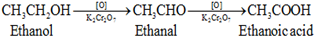(c) Substitution reaction: These reactions involve substituent of one or more part of molecule in the presence of appropriate reagent. For example, reaction of methane with chlorine gas in the presence of sunlight substitute hydrogen atoms of methane with chlorine atom and form methyl chloride.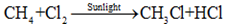(d) Saponification reaction: It is hydrolysis of ester in the presence of strong alkali like sodium hydroxide. It results in the formation of sodium salts of carboxylic acid and alcohol.$CH_{3}COOCH_{3} + NaOH → CH_{3}COONa + CH_{3}OH$(e) Combustion reaction: Burning of organic compounds in the presence of air forms carbon dioxide and water vapour. This reaction is called as combustion reaction.$CH_{4} + 2O_{2} → CO_{2} + 2H_{2}O + Heat and light$It is an exothermic reaction and releases a large amount of energy in the form of heat. Q.57. An organic compound A on heating with concentrated$H_{2}SO_{4}$forms a compound B which on addition of one mole of hydrogen in presence of Ni forms a compound C. One mole of compound C on combustion forms two moles of$CO_{2}$and three moles of$H_{2}O$. Identify the compounds A, B and C and write the chemical equation of the reactions involved. Answer. Combustion of compound C forms two moles of$CO_{2}$and three moles of$H_{2}O$so it must contain two carbon atoms and six hydrogen atoms with molecular formula$C_{2}H_{6}$that is ethane. Ethane is formed by the addition of one mole of hydrogen in the presence of Ni catalyst on compound B. So compound B must be unsaturated hydrocarbon with two carbon atom that is ethene$(CH_{2}=CH_{2})$.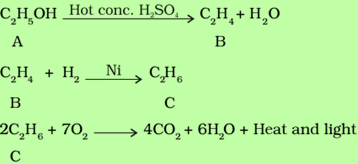Hence; A = Ethanol$(CH_{3}CH_{2}OH), B = Ethene (CH_{2} = CH_{2})$and C = Ethane (CH_{3} ─ CH_{3})$